# Chapter 12 Using Colors in Plots

In ggplot2’s implementation of the grammar of graphics, `color` is an aesthetic, just like x position, y position, and `size`. If color is just another aesthetic, why does it deserve its own chapter? The reason is that color is a more complicated aesthetic than the others. Instead of simply moving geoms left and right or making them larger and smaller, when you use color, there are many degrees of freedom and many more choices to make. What palette should you use for discrete values? Should you use a gradient with several different hues? How do you choose colors that can be interpreted accurately by those with color-vision deficiencies? In this chapter, I’ll address these issues.

## 12.1 Setting the Colors of Objects

### 12.1.1 Problem

You want to set the color of some geoms in your graph.

### 12.1.2 Solution

In the call to the geom, set the values of `colour` or `fill` (Figure 12.1):

``````library(MASS)  # Load MASS for the birthwt data set

ggplot(birthwt, aes(x = bwt)) +
geom_histogram(fill = "red", colour = "black")

ggplot(mtcars, aes(x = wt, y = mpg)) +
geom_point(colour = "red")``````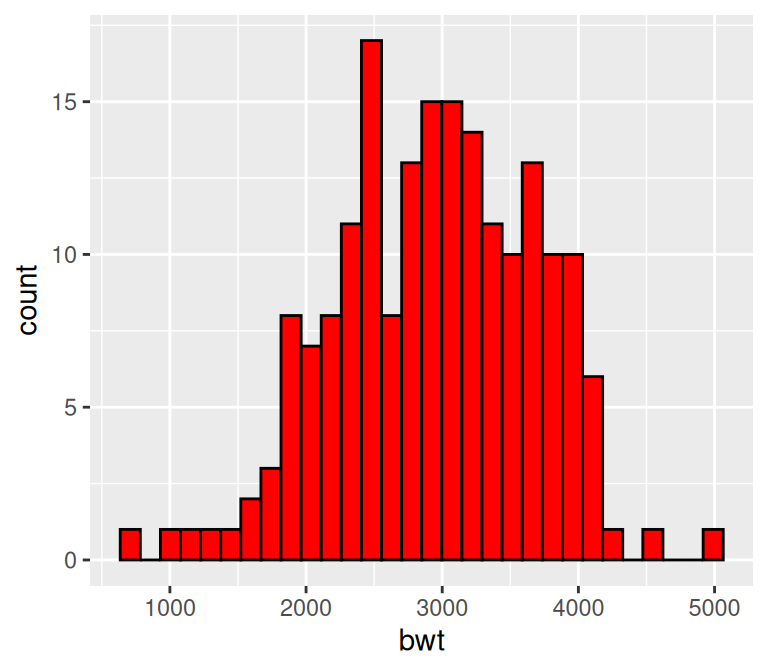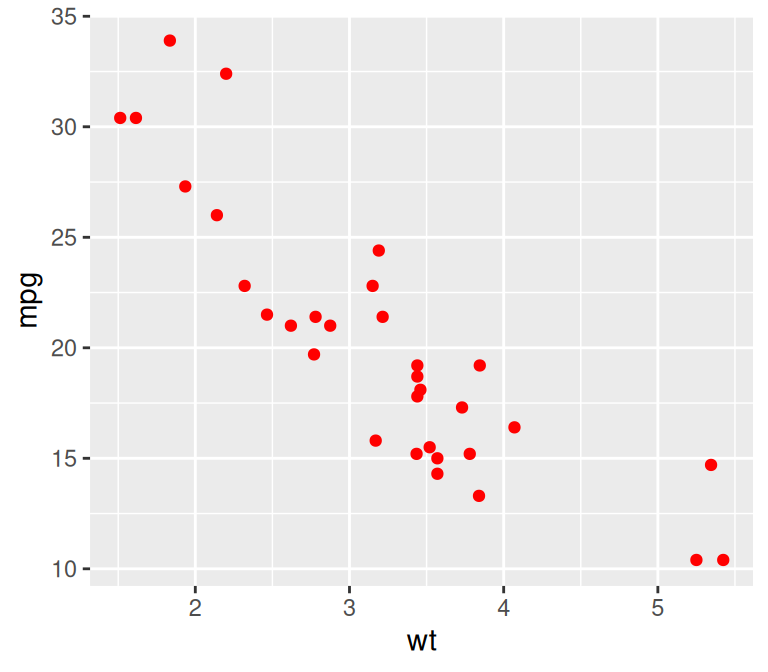Figure 12.1: Setting `fill` and `colour` (left); Setting `colour` for points (right)

### 12.1.3 Discussion

In ggplot2, there’s an important difference between setting and mapping aesthetic properties. In the preceding example, we set the color of the objects to “red”.

Generally speaking, `colour` controls the color of lines and of the outlines of polygons, while `fill` controls the color of the fill area of polygons. However, point shapes are sometimes a little different. For most point shapes, the color of the entire point is controlled by `colour`, not `fill`. The exception is the point shapes (21–25) that have both a fill and an outline.

You can use `colour` or `color` interchangeably with ggplot2. In this book, I’ve used `colour`, in keeping with the form used in the official ggplot2 documentation.

See Recipe 12.5 for more on specifying colors.

## 12.2 Representing Variables with Colors

### 12.2.1 Problem

You want to use a variable (column from a data frame) to control the color of geoms.

### 12.2.2 Solution

In the call to the geom, inside of `aes()`, set the value of `colour` or `fill` to the name of one of the columns in the data (Figure 12.2):

``````library(gcookbook)  # Load gcookbook for the cabbage_exp data set

# These both have the same effect
ggplot(cabbage_exp, aes(x = Date, y = Weight, fill = Cultivar)) +
geom_col(colour = "black", position = "dodge")

ggplot(cabbage_exp, aes(x = Date, y = Weight)) +
geom_col(aes(fill = Cultivar), colour = "black", position = "dodge")

# These both have the same effect
ggplot(mtcars, aes(x = wt, y = mpg, colour = cyl)) +
geom_point()

ggplot(mtcars, aes(x = wt, y = mpg)) +
geom_point(aes(colour = cyl))``````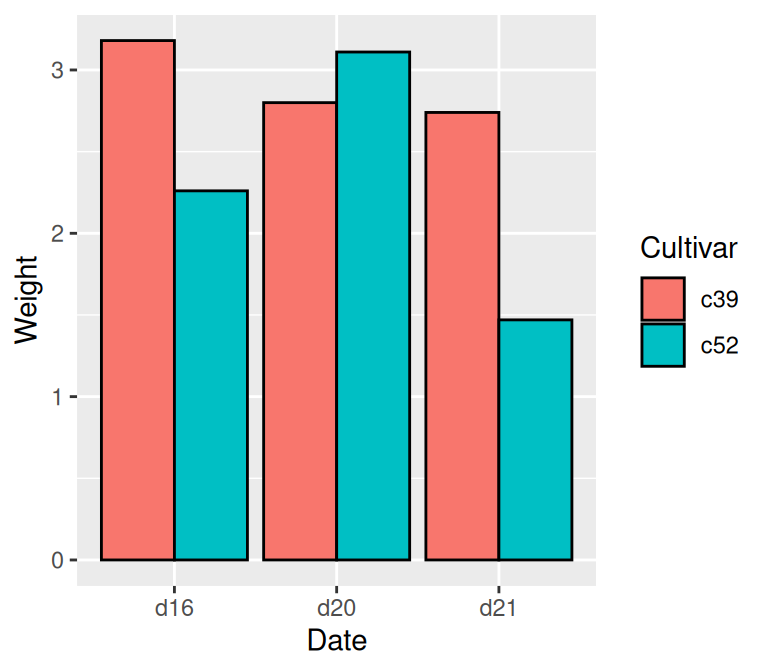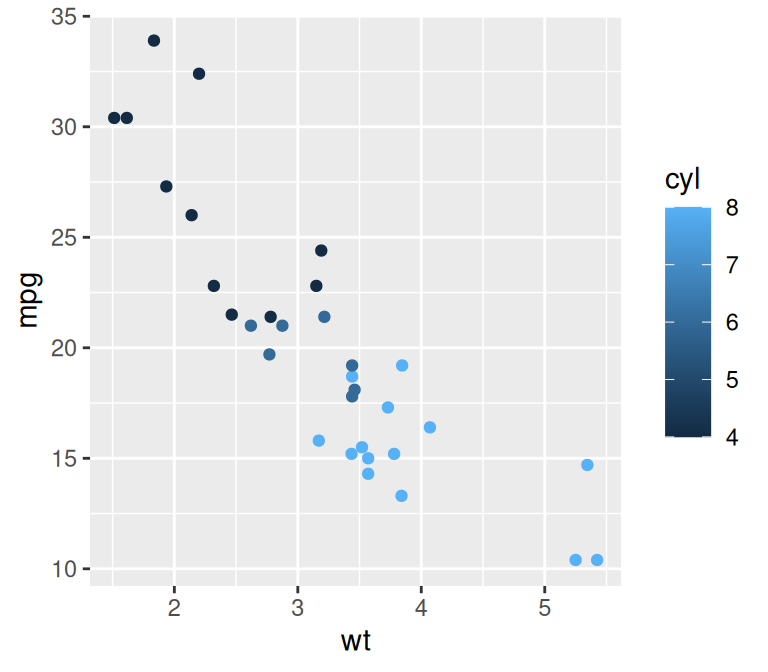Figure 12.2: Mapping a variable to `fill` (left); Mapping a variable to `colour` for points (right)

When the mapping is specified in `ggplot()` it is used as the default mapping, which is inherited by all the geoms. Within a geom, the default mappings can be overridden.

### 12.2.3 Discussion

In the `cabbage_exp` example, the variable `Cultivar` is mapped to `fill`. The `Cultivar` column in `cabbage_exp` is a factor, so ggplot treats it as a categorical variable. You can check the type using `str()`:

``````str(cabbage_exp)
#> 'data.frame':    6 obs. of  6 variables:
#>  \$ Cultivar: Factor w/ 2 levels "c39","c52": 1 1 1 2 2 2
#>  \$ Date    : Factor w/ 3 levels "d16","d20","d21": 1 2 3 1 2 3
#>  \$ Weight  : num  3.18 2.8 2.74 2.26 3.11 1.47
#>  \$ sd      : num  0.957 0.279 0.983 0.445 0.791 ...
#>  \$ n       : int  10 10 10 10 10 10
#>  \$ se      : num  0.3025 0.0882 0.311 0.1408 0.2501 ...``````

In the `mtcars` example, `cyl` is numeric, so it is treated as a continuous variable. Because of this, even though the actual values of `cyl` include only 4, 6, and 8, the legend has entries for the intermediate values 5 and 7. To make ggplot treat `cyl` as a categorical variable, you can convert it to a factor in the call to `ggplot()` (Figure 12.3, left), or you can modify the data so that the column is a character vector or factor (Figure 12.3, right):

``````# Convert to factor in call to ggplot()
ggplot(mtcars, aes(x = wt, y = mpg, colour = factor(cyl))) +
geom_point()

# Another method: Convert to factor in the data
library(dplyr)
mtcars_mod <- mtcars %>%
mutate(cyl = as.factor(cyl))  # Convert cyl to a factor

ggplot(mtcars_mod, aes(x = wt, y = mpg, colour = cyl)) +
geom_point()``````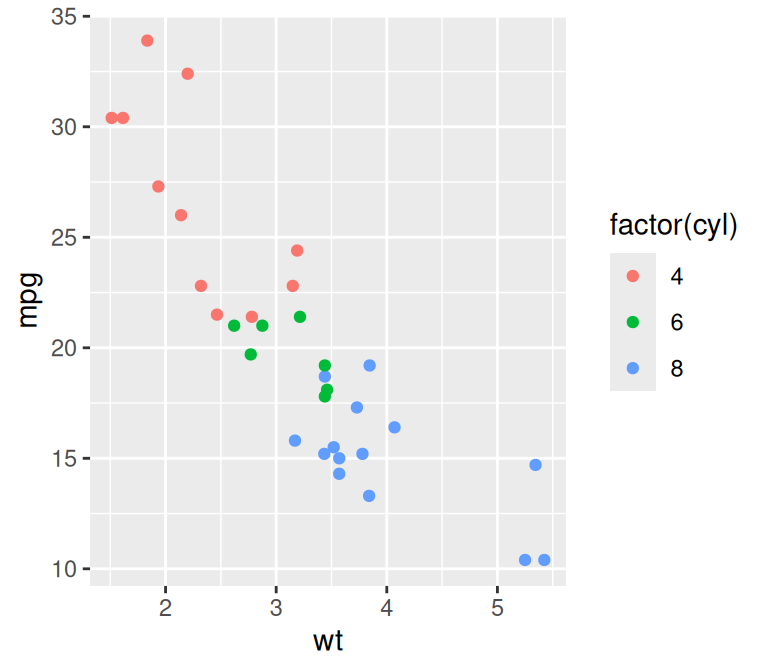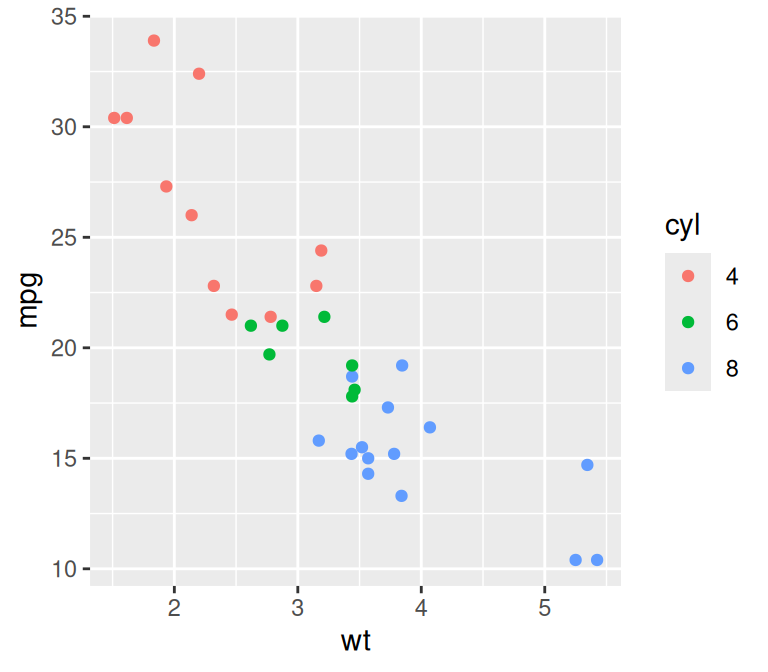Figure 12.3: Converting `cyl` to a factor, within the call to ggplot (left); By modifying the dataframe (right)

You may also want to change the colors that are used in the scale. For continuous data, see Recipe 12.6. For discrete data, see Recipe 12.4 and Recipe 12.5.

## 12.3 Using a Colorblind-Friendly Palette

### 12.3.1 Problem

You want to select a color palette that can also be distinguished by colorblind viewers.

### 12.3.2 Solution

Use the color scales in the viridis package.

The viridis package contains a set of beautiful color scales that are each designed to span as wide a palette as possible, making it easier to see differences in your data. These scales are also designed to be perceptually uniform, printable in grey scale, and easier to read by those with colorblindness.

Here is an example from the introduction page to viridis (https://cran.r-project.org/web/packages/viridis/vignettes/intro-to-viridis.html; Figure 12.4):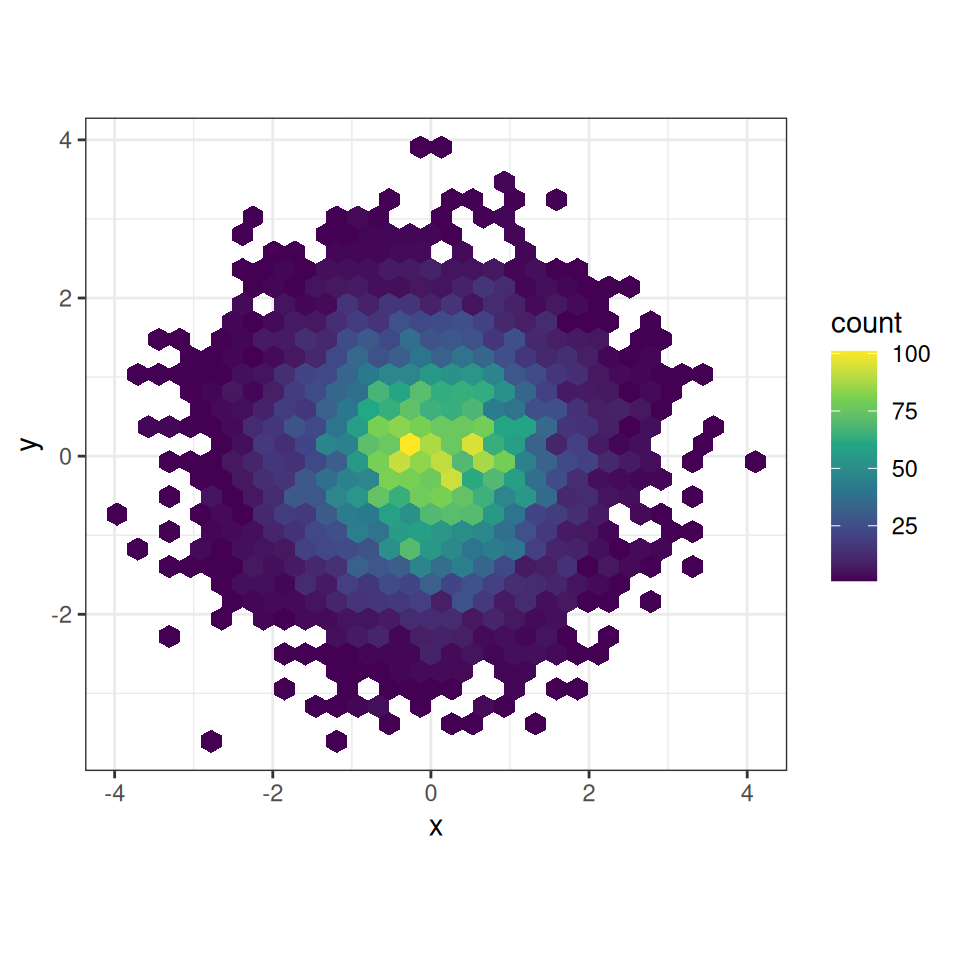Figure 12.4: Example of viridis color palette

The viridis color scales can be implemented for data that is both continuous and discrete in nature. You will need to add `scale_fill_viridis_c()` to your plot if your data is continuous. If your data is discrete you will need to use `scale_fill_viridis_d()` instead, as in Figure 12.5 below:

``````library(gcookbook)  # Load gcookbook for the uspopage data set

# Create the base plot
uspopage_plot <- ggplot(uspopage, aes(x = Year, y = Thousands, fill = AgeGroup)) +
geom_area()

# Add the viridis color scale
uspopage_plot +
scale_fill_viridis_d()``````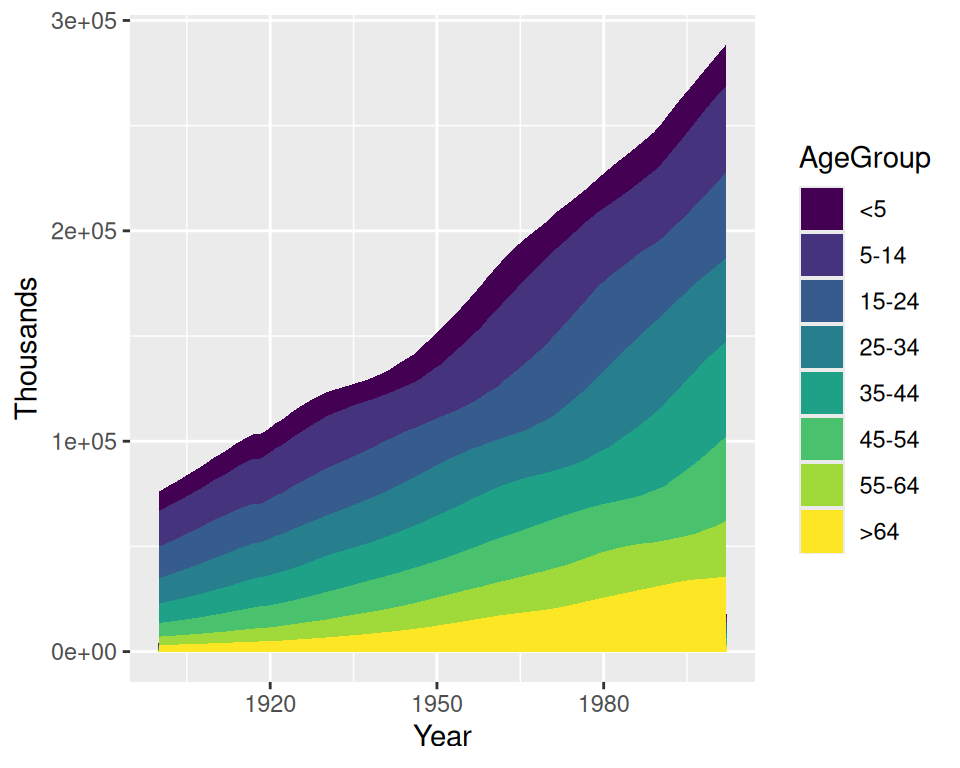Figure 12.5: A plot with the colorblind-friendly viridis palette

### 12.3.3 Discussion

About 8 percent of males and 0.5 percent of females have some form of color-vision deficiency, so there’s a good chance that someone in your audience will be among them. There are many different forms of color blindness - the palettes that are mentioned in this book are designed to enable people with any of the most common forms of color-vision deficiency to distinguish the colors. (Monochromacy, or total colorblindness, is rare. Those who have it can only see differences in brightness.)

The viridis color scales come with the current version of ggplot2 (3.0.0). There are also other color palettes that are friendly to users with color blindness, such as those in cetcolor package (see below).

To see more on the different viridis palettes, see `?scales::viridis_pal`.

The cetcolor scales: https://github.com/coatless/cetcolor.

The Color Oracle program (http://colororacle.org) can simulate how things on your screen appear to someone with color vision deficiency, but keep in mind that the simulation isn’t perfect. In my informal testing, I viewed an image with simulated red-green deficiency, and I could distinguish the colors just fine – but others with actual red-green deficiency viewed the same image and couldn’t tell the colors apart!

## 12.4 Using a Different Palette for a Discrete Variable

### 12.4.1 Problem

You want to use different colors for a discrete mapped variable.

### 12.4.2 Solution

Use one of the scales listed in Table 12.1.

Table 12.1: Discrete fill and color scales
Fill scale Color scale Description
`scale_fill_discrete()` `scale_colour_discrete()` Colors evenly spaced around the color wheel (same as `hue`)
`scale_fill_hue()` `scale_colour_hue()` Colors evenly spaced around the color wheel (same as `discrete`)
`scale_fill_grey()` `scale_colour_grey()` Greyscale palette
`scale_fill_viridis_d()` `scale_colour_viridis_d()`
Viridis palettes
`scale_fill_brewer()` `scale_colour_brewer()` ColorBrewer palettes
`scale_fill_manual()` `scale_colour_manual()` Manually specified colors

In the example here we’ll use the default palette (hue), a viridis palette, and a ColorBrewer palette (Figure 12.6):

``````library(gcookbook)  # Load gcookbook for the uspopage data set
library(viridis)  # Load viridis for the viridis palette

# Create the base plot
uspopage_plot <- ggplot(uspopage, aes(x = Year, y = Thousands, fill = AgeGroup)) +
geom_area()

# These four specifications all have the same effect
uspopage_plot
# uspopage_plot + scale_fill_discrete()
# uspopage_plot + scale_fill_hue()
# uspopage_plot + scale_color_viridis()

# Viridis palette
uspopage_plot +
scale_fill_viridis(discrete = TRUE)

# ColorBrewer palette
uspopage_plot +
scale_fill_brewer()``````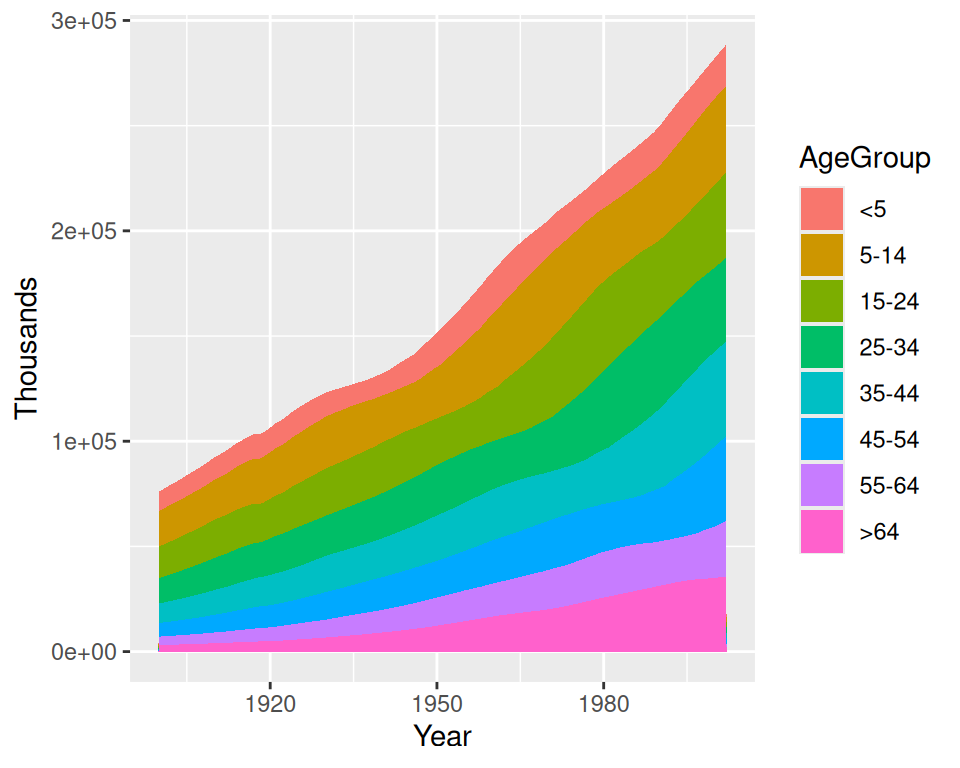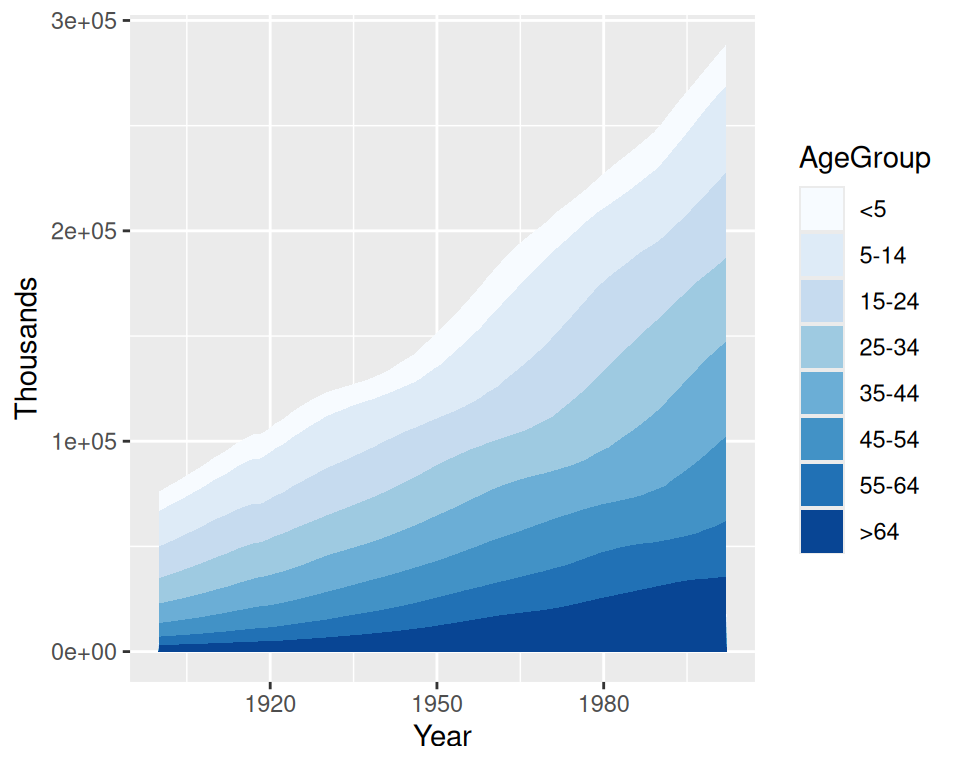Figure 12.6: Default palette (using hue; top); A viridis palette (middle); A ColorBrewer palette (bottom)

### 12.4.3 Discussion

Changing a palette is a modification of the color (or fill) scale: it involves a change in the mapping from numeric or categorical values to aesthetic attributes. There are two types of scales that use colors: fill scales and color scales.

With `scale_fill_hue()`, the colors are taken from around the color wheel in the HCL (hue-chroma-lightness) color space. The default lightness value is 65 on a scale from 0–100. This is good for filled areas, but it’s a bit light for points and lines. To make the colors darker for points and lines, as in Figure 12.7 (right), set the value of `l` (luminance/lightness):

``````# Create the base scatter plot
hw_splot <- ggplot(heightweight, aes(x = ageYear, y = heightIn, colour = sex)) +
geom_point()

# Default lightness = 65
hw_splot

# Slightly darker, set lightness = 45
hw_splot +
scale_colour_hue(l = 45)``````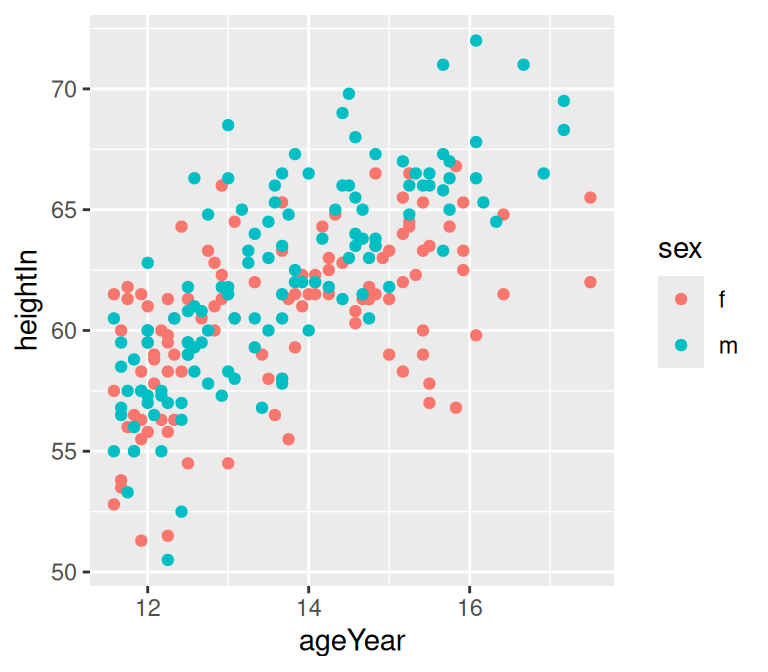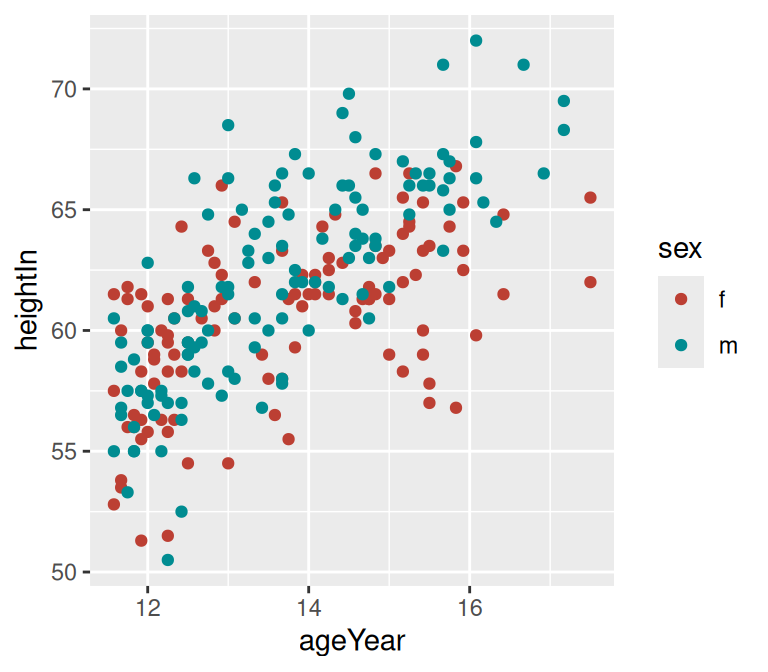Figure 12.7: Points with default lightness (left); With lightness set to 45 (right)

The viridis package provides a number of color scales that make it easy to see differences across your data. See Recipe 12.3 for more details and examples.

The ColorBrewer package provides a number of palettes. You can generate a graphic showing all of them, as shown in Figure 12.8: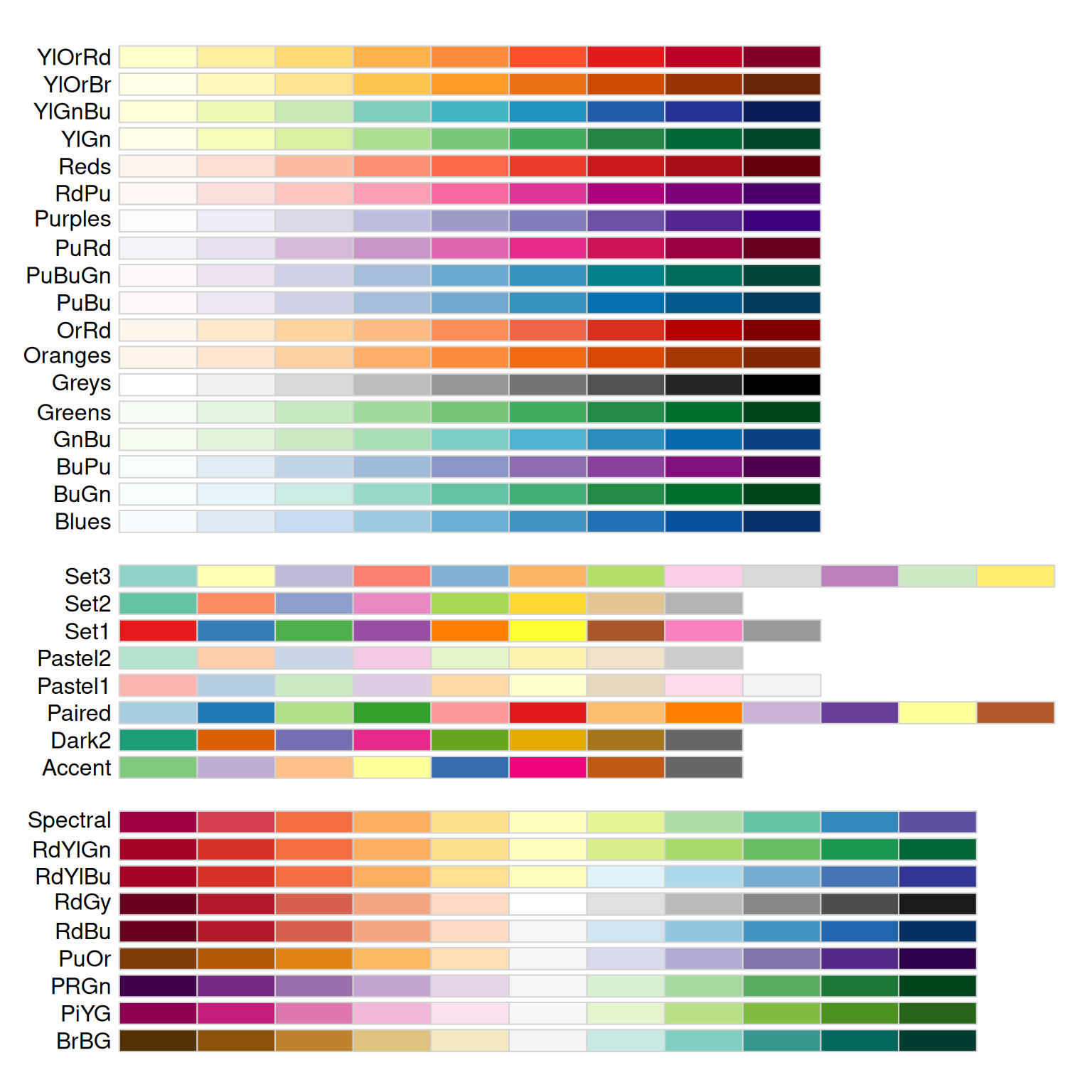Figure 12.8: All the ColorBrewer palettes

``````library(RColorBrewer)
display.brewer.all()``````

The ColorBrewer palettes can be selected by name. For example, this will use the “Oranges” palette (Figure 12.9):

``````hw_splot +
scale_colour_brewer(palette = "Oranges") +
theme_bw()``````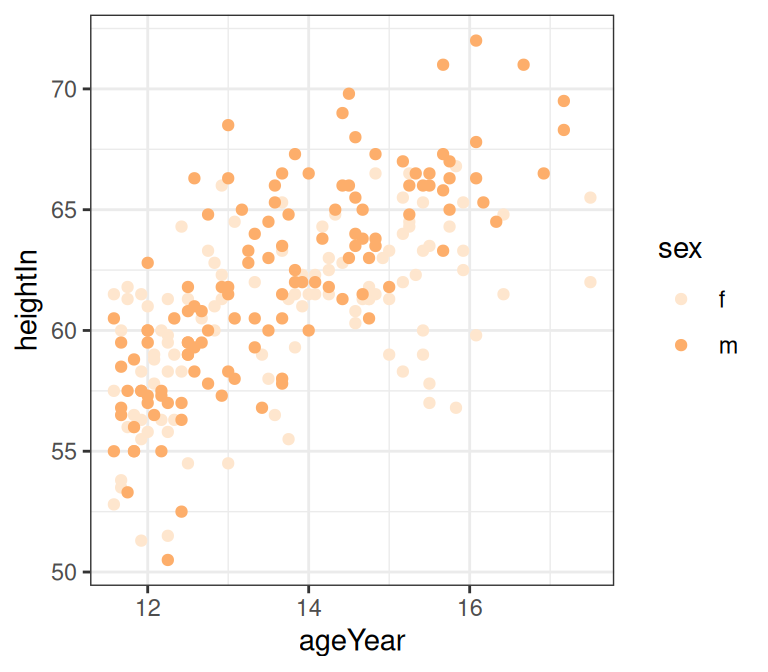Figure 12.9: Using a named ColorBrewer palette

You can also use a palette of greys. This is useful for print when the output is in black and white. The default is to start at 0.2 and end at 0.8, on a scale from 0 (black) to 1 (white), but you can change the range, as shown in Figure 12.10.

``````hw_splot +
scale_colour_grey()

# Reverse the direction and use a different range of greys
hw_splot +
scale_colour_grey(start = 0.7, end = 0)``````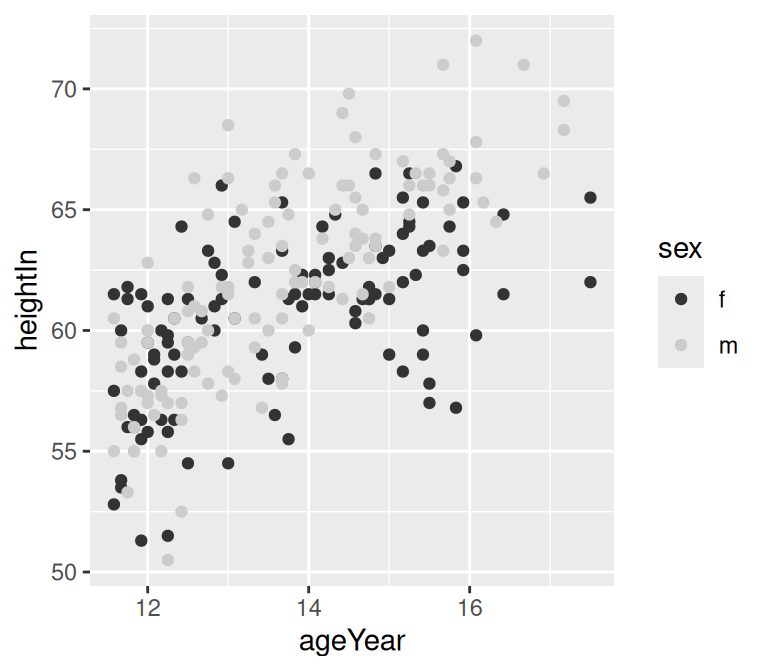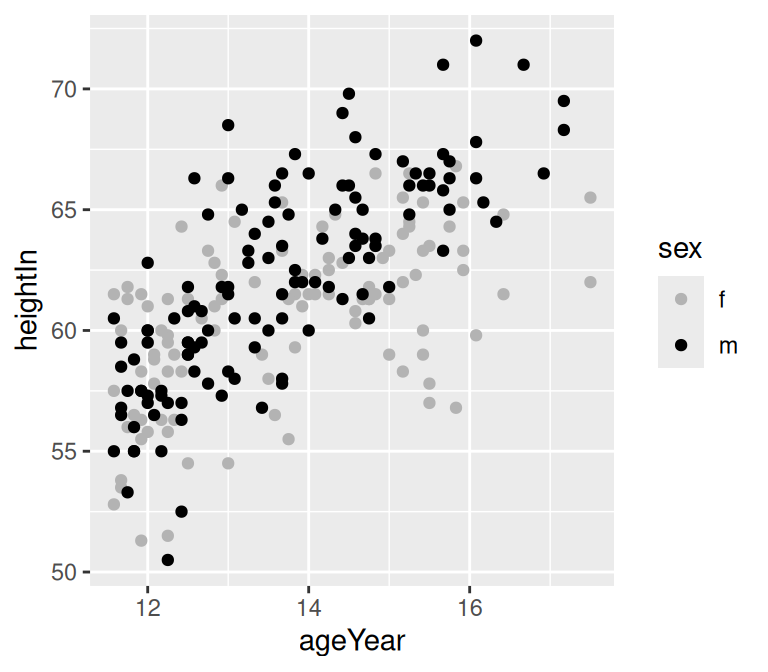Figure 12.10: Using the default grey palette (left); A different grey palette (right)

See Recipe 10.4 for more information about reversing the legend.

To select colors manually, see Recipe 12.5.

For more about viridis, see https://cran.r-project.org/web/packages/viridis/vignettes/intro-to-viridis.html. For more about ColorBrewer, see http://colorbrewer2.org.

## 12.5 Using a Manually Defined Palette for a Discrete Variable

### 12.5.1 Problem

You want to use different colors for a discrete mapped variable.

### 12.5.2 Solution

In the example here, we’ll manually define colors by specifying values with `scale_colour_manual()` (Figure 12.11). The colors can be named, or they can be specified with RGB values:

``````library(gcookbook)  # Load gcookbook for the heightweight data set

# Create the base plot
hw_plot <- ggplot(heightweight, aes(x = ageYear, y = heightIn, colour = sex)) +
geom_point()

# Using color names
hw_plot +
scale_colour_manual(values = c("red", "blue"))

# Using RGB values
hw_plot +
scale_colour_manual(values = c("#CC6666", "#7777DD"))

# Using RGB values based on the viridis color scale
hw_plot +
scale_colour_manual(values = c("#440154FF", "#FDE725FF")) +
theme_bw()``````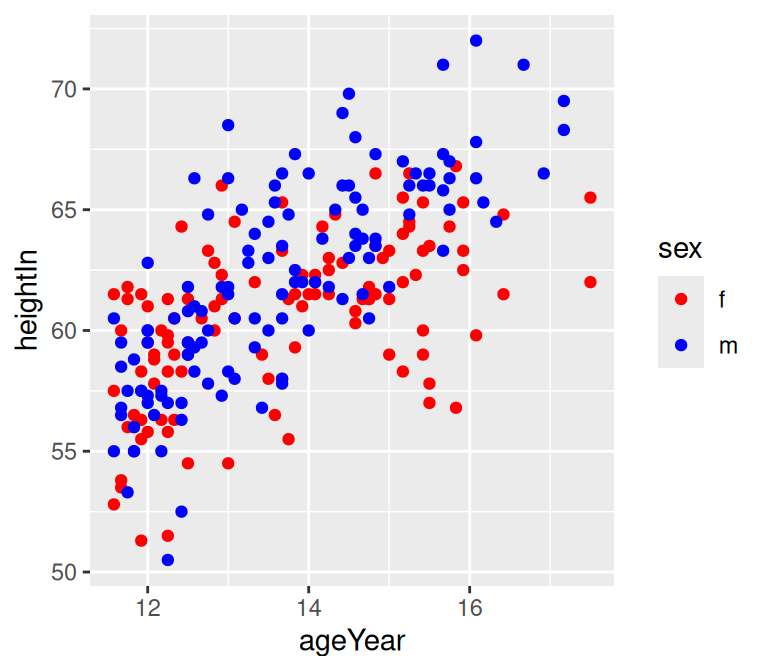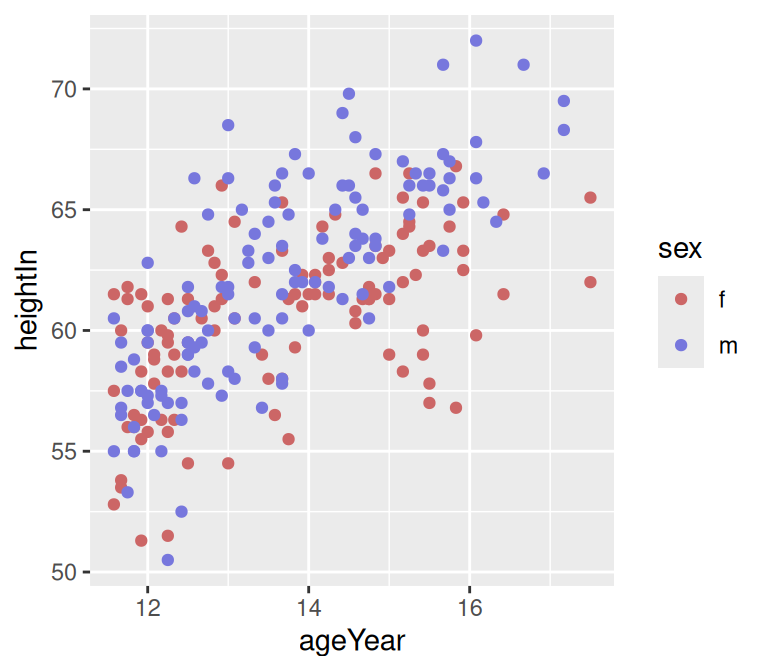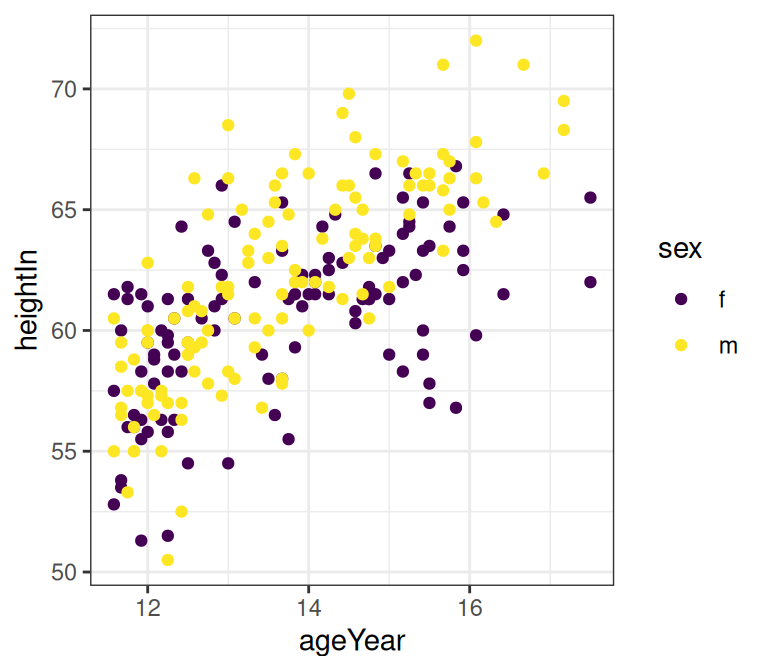Figure 12.11: Scatter plot with named colors (top left); With slightly different RGB colors (top right); With colors from the viridis color scale (bottom)

For fill scales, use `scale_fill_manual()` instead.

### 12.5.3 Discussion

The order of the items in the values vector matches the order of the factor levels for the discrete scale. In the preceding example, the order of sex is f, then m, so the first item in values goes with f and the second goes with m. Here’s how to see the order of factor levels:

``````levels(heightweight\$sex)
#>  "f" "m"``````

If the variable is a character vector, not a factor, it will automatically be converted to a factor, and by default the levels will appear in alphabetical order.

It’s possible to specify the colors in a different order by using a named vector:

``````hw_plot +
scale_colour_manual(values = c(m = "blue", f = "red"))``````

There is a large set of named colors in R, which you can see by running `color()`. Some basic color names are useful: “white”, “black”, “grey80”, “red”, “blue”, “darkred”, and so on. There are many other named colors, but their names are generally not very informative (I certainly have no idea what “thistle3” and “seashell” look like), so it’s often easier to use numeric RGB values for specifying colors.

RGB colors are specified as six-digit hexadecimal (base-16) numbers of the form `#RRGGBB`. In hexadecimal, the digits go from 0 to 9, and then continue with A (10 in base 10) to F (15 in base 10). Each color is represented by two digits and can range from 00 to FF (255 in base 10). So, for example, the color `#FF0099` has a value of 255 for red, 0 for green, and 153 for blue, resulting in a shade of magenta. The hexadecimal numbers for each color channel often repeat the same digit because it makes them a little easier to read, and because the precise value of the second digit has a relatively insignificant effect on appearance.

Here are some rules of thumb for specifying and adjusting RGB colors:

• In general, higher numbers are brighter and lower numbers are darker.
• To get a shade of grey, set all the channels to the same value.
• The opposites of RGB are CMY: Cyan, Magenta, and Yellow. Higher values for the red channel make it more red, and lower values make it more cyan. The same is true for the pairs green and magenta, and blue and yellow.

You may want to manually select colors based on the color scales in the viridis package, as described in Recipe 12.4. You can do so by calling `viridis()` and passing it the number of discrete categories you have. This will generate the RGB hexadecimal values. You can similarly generate the RGB values for the other color scales in the viridis package: “magma”, “plasma”, “inferno”, and “cividis”.

``````library(viridis)
viridis(2)  ## Specifying 2 discrete categories with the viridis color scale
#>  "#440154FF" "#FDE725FF"
inferno(5)  ## Specifying 5 discrete categories with the inferno color scale
#>  "#000004FF" "#56106EFF" "#BB3754FF" "#F98C0AFF" "#FCFFA4FF"``````

A chart of RGB color codes: http://html-color-codes.com.

## 12.6 Using a Manually Defined Palette for a Continuous Variable

### 12.6.1 Problem

You want to use different colors for a continuous variable.

### 12.6.2 Solution

In the example here, we’ll specify the colors for a continuous variable using various gradient scales (Figure 12.12). The colors can be named, or they can be specified with RGB values:

``````library(gcookbook)  # Load gcookbook for the heightweight data set

# Create the base plot
hw_plot <- ggplot(heightweight, aes(x = ageYear, y = heightIn, colour = weightLb)) +
geom_point(size = 3)

hw_plot

# A gradient with a white midpoint
library(scales)
hw_plot +
low = muted("red"),
mid = "white",
high = muted("blue"),
midpoint = 110
)

# With a gradient between two colors (black and white)
hw_plot +
scale_colour_gradient(low = "black", high = "white")

# A gradient of n colors
hw_plot +
scale_colour_gradientn(colours = c("darkred", "orange", "yellow", "white"))``````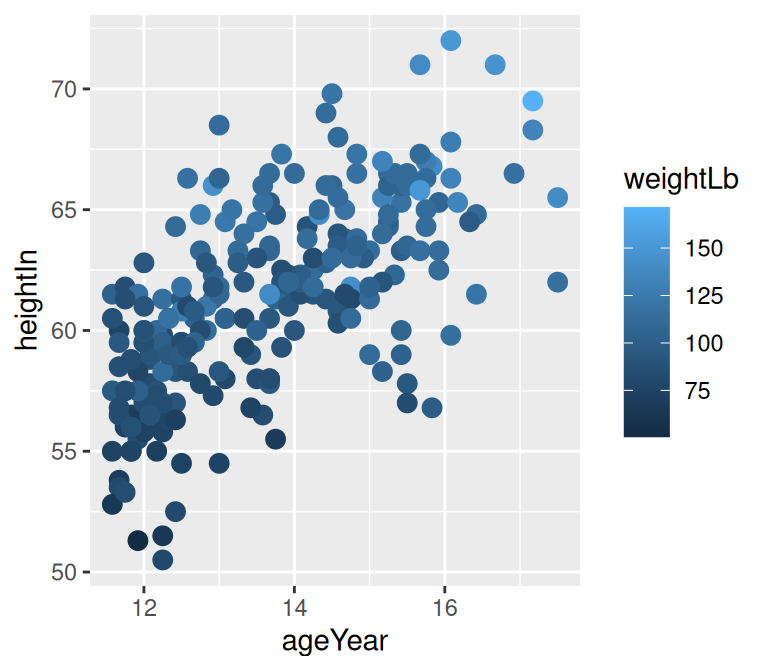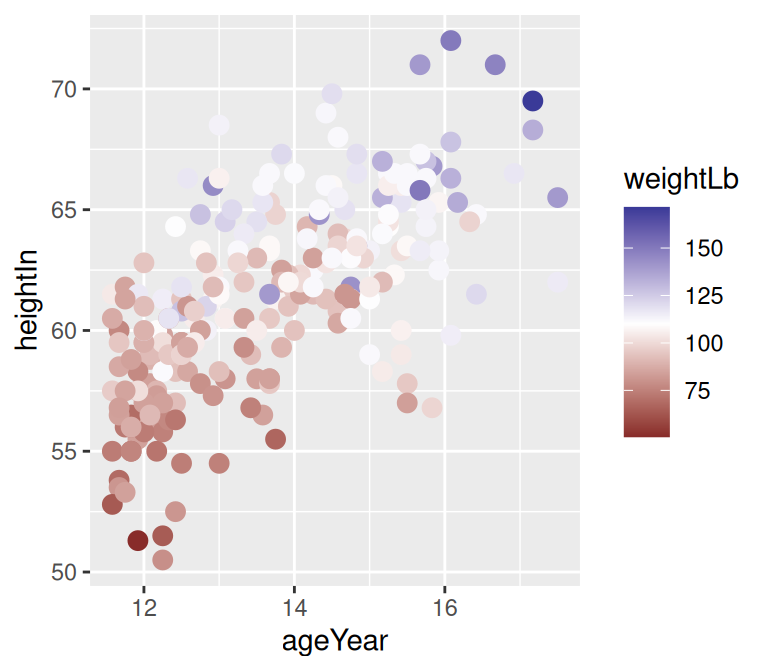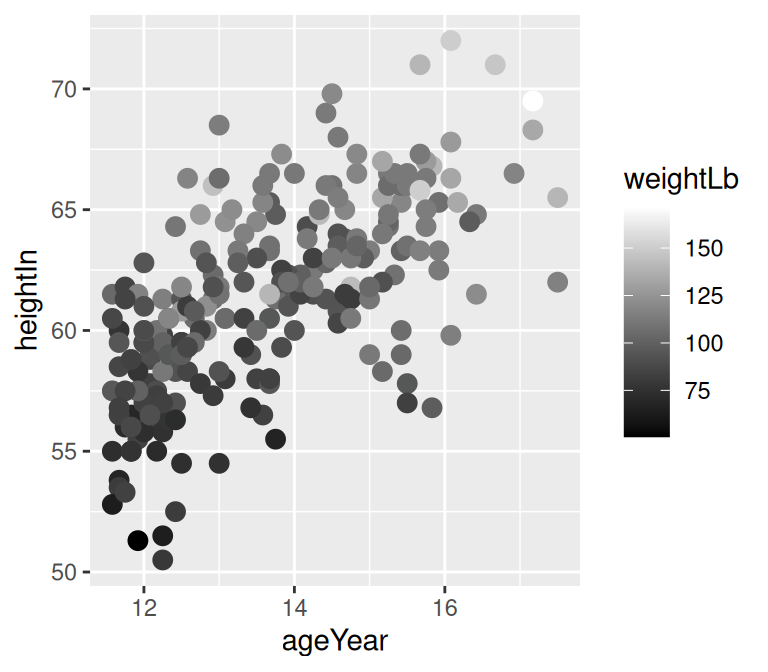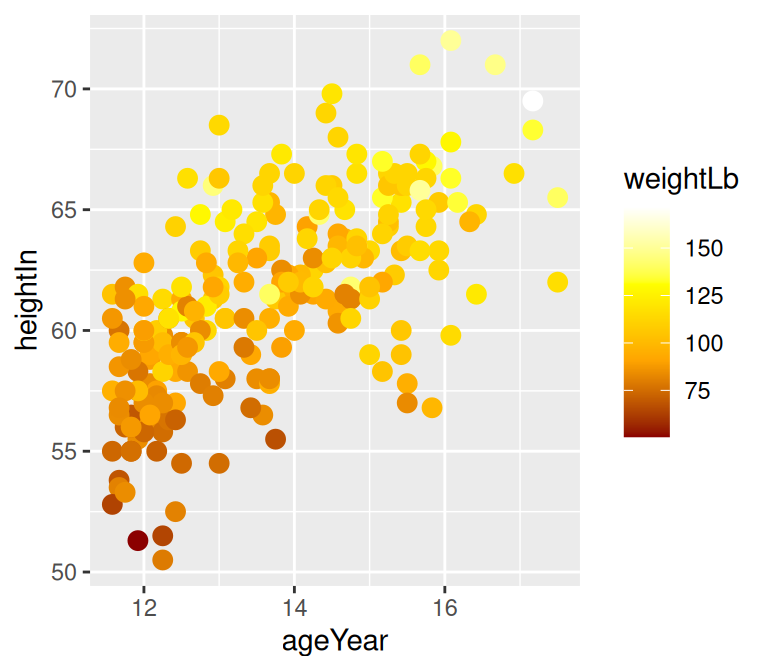Figure 12.12: Clockwise from top left: default colors, two-color gradient (black and white) with `scale_colour_gradient()`, three-color gradient with midpoint with `scale_colour_gradient2()`, four-color gradient with `scale_colour_gradientn()`

For fill scales, use `scale_fill_xxx()` versions instead, where `xxx` is one of `gradient`, `gradient2`, or `gradientn`.

### 12.6.3 Discussion

Mapping continuous values to a color scale requires a continuously changing palette of colors. Table 12.2. lists the continuous color and fill scales.

Table 12.2: Continuous fill and color scales
Fill scale Color scale Description
`scale_fill_gradient()` `scale_colour_gradient()` Two-color gradient
`scale_fill_gradient2()` `scale_colour_gradient2()` Gradient with a middle color and two colors that diverge from it
`scale_fill_gradientn()` `scale_colour_gradientn()` Gradient with n colors, equally spaced
`scale_fill_viridis_c()` `scale_colour_viridis_c()` Viridis palettes

Notice that we used the `muted()` function in the examples. This is a function from the scales package that returns an RGB value that is a less-saturated version of the color chosen.

If you want use a discrete (categorical) scale instead of a continuous one, you can recode your data into categorical values. See Recipe 15.14.

## 12.7 Coloring a Shaded Region Based on Value

### 12.7.1 Problem

You want to set the color of a shaded region based on the y value.

### 12.7.2 Solution

Add a column that categorizes the y values, then map that column to fill. In this example, we’ll first categorize the values as positive or negative:

``````library(gcookbook)  # Load gcookbook for the climate data set
library(dplyr)

climate_mod <- climate %>%
filter(Source == "Berkeley") %>%
mutate(valence = if_else(Anomaly10y >= 0, "pos", "neg"))

climate_mod
#>       Source Year Anomaly1y Anomaly5y Anomaly10y Unc10y valence
#> 1   Berkeley 1800        NA        NA     -0.435  0.505     neg
#> 2   Berkeley 1801        NA        NA     -0.453  0.493     neg
#> 3   Berkeley 1802        NA        NA     -0.460  0.486     neg
#>  ...<199 more rows>...
#> 203 Berkeley 2002        NA        NA      0.856  0.028     pos
#> 204 Berkeley 2003        NA        NA      0.869  0.028     pos
#> 205 Berkeley 2004        NA        NA      0.884  0.029     pos``````

Once we’ve categorized the values as positive or negative, we can make the plot, mapping valence to the fill color, as shown in Figure 12.13:

``````ggplot(climate_mod, aes(x = Year, y = Anomaly10y)) +
geom_area(aes(fill = valence)) +
geom_line() +
geom_hline(yintercept = 0)``````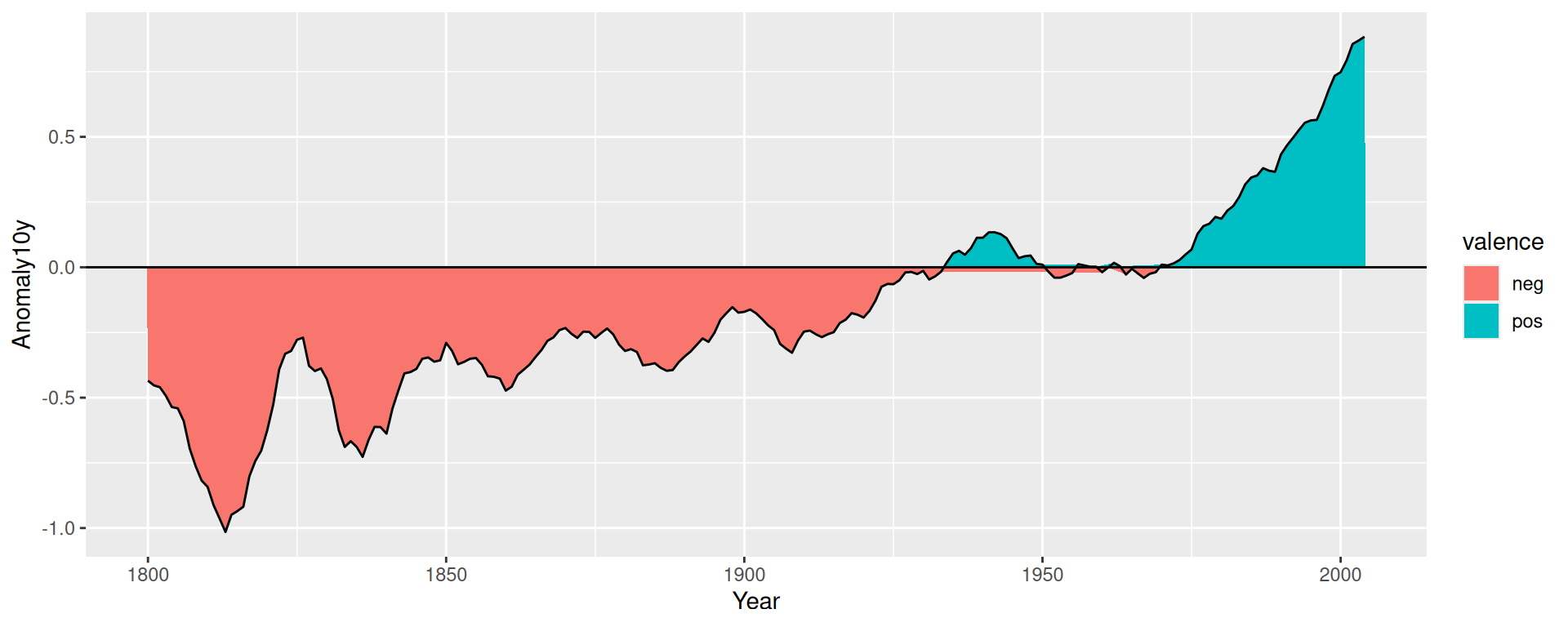Figure 12.13: Mapping valence to fill color-notice the red area under the zero line around 1950

### 12.7.3 Discussion

If you look closely at the figure, you’ll notice that there are some stray shaded areas near the zero line. This is because each of the two colored areas is a single polygon bounded by the data points, and the data points are not actually at zero. To solve this problem, we can interpolate the data to 1,000 points by using `approx()`:

``````# approx() returns a list with x and y vectors
interp <- approx(climate_mod\$Year, climate_mod\$Anomaly10y, n = 1000)

# Put in a data frame and recalculate valence
cbi <- data.frame(Year = interp\$x, Anomaly10y = interp\$y) %>%
mutate(valence = if_else(Anomaly10y >= 0, "pos", "neg"))``````

It would be more precise (and more complicated) to interpolate exactly where the line crosses zero, but `approx()` works fine for the purposes here.

Now we can plot the interpolated data (Figure 12.14). This time we’ll make a few adjustments – we’ll make the shaded regions partially transparent, change the colors, remove the legend, and remove the padding on the left and right sides:

``````ggplot(cbi, aes(x = Year, y = Anomaly10y)) +
geom_area(aes(fill = valence), alpha = .4) +
geom_line() +
geom_hline(yintercept = 0) +
scale_fill_manual(values = c("#CCEEFF", "#FFDDDD"), guide = FALSE) +
scale_x_continuous(expand = c(0, 0))``````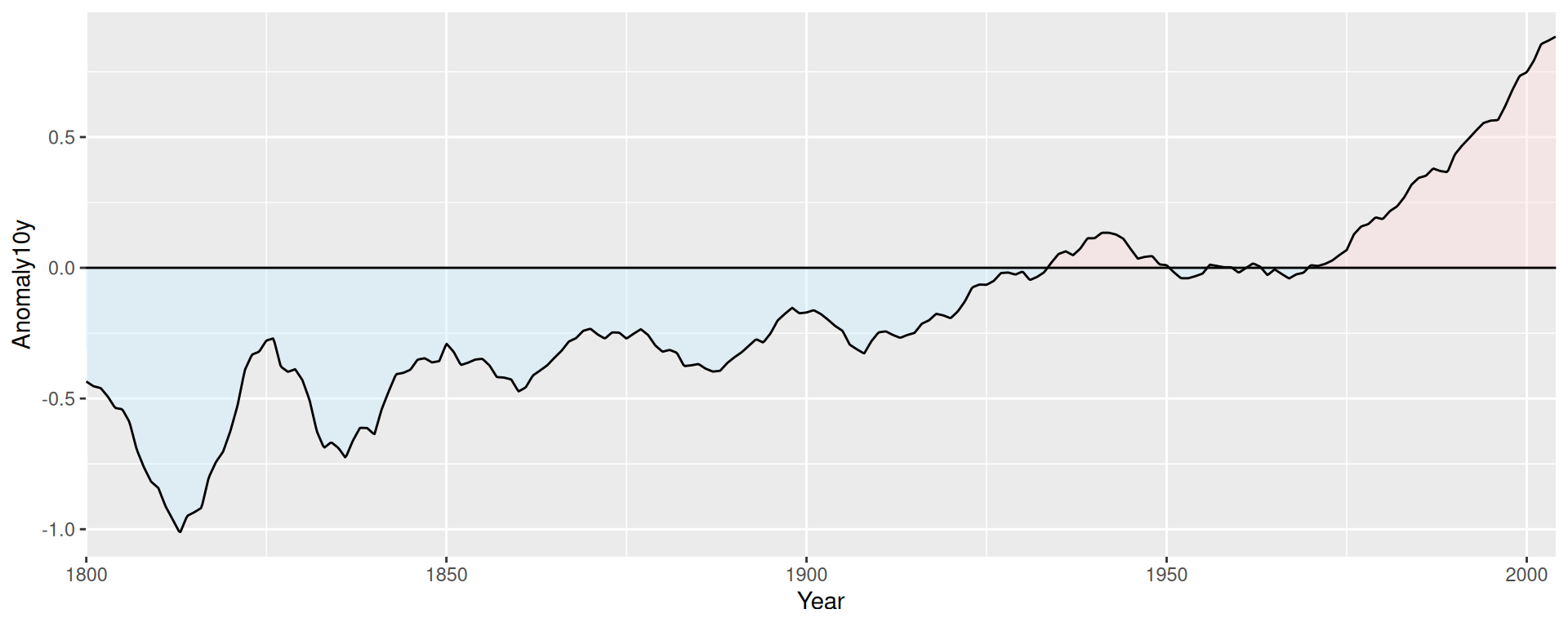Figure 12.14: Shaded regions with interpolated data